# Basic Geometry : How to find the area of a square

## Example Questions

← Previous 1 3 4 5 6 7 8 9 15 16

### Example Question #1 : How To Find The Area Of A Square

The sides of a square garden are 10 feet long. What is the area of the garden?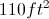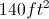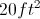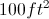Explanation:

The formula for the area of a square is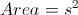where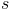is the length of the sides. So the solution can be found by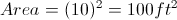### Example Question #1 : How To Find The Area Of A Square

The length of line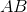in the figure below is 15 inches. What is the area of the square?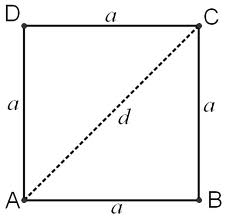Cannot be determined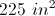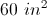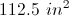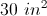Explanation:

Sinceis a square, the sideis the same length as all of the other three sides.

We get area by multiplying length by width (or base by height, if you prefer), since all sides are equal, it looks like this: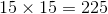Don't forget, the units are SQUARE INCHES.

### Example Question #1 : How To Find The Area Of A Square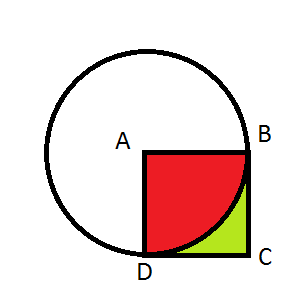Point A is the center of the circle.

Figure ABCD is a square.

The radius of the circle isunits.

Find the area of the green-colored shape.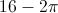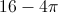Explanation:

Square ABCD contains both the red and green shapes. The red shape is equal to the area of one-fourth of the circle. Finding the area of square ABCD and subtracting only the area of the red shape will give the area of only the green shape.

Since ABCD is a square, angle BAC is a right angle that sits at the center of the circle (point A). Since a right angle is 90o and a circle is 360o, the red shape's area must be one quarter (or) of the entire circle's area. Use the equation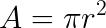to find the area of the entire circle, then multiply this byto find the area of only the red shape.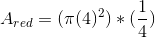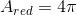Subtracting this from the area of the square gives the area of the green area outside of the circle.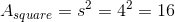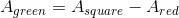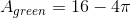### Example Question #4 : How To Find The Area Of A Square

What is the area of the following square if the length of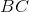is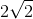?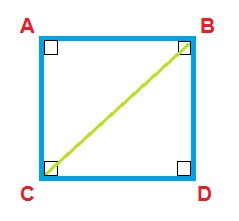1

4

5

3

2

4

Explanation: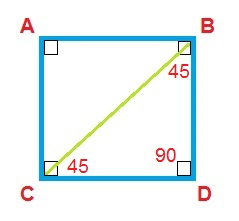We need to find the length of the side of the square in order to find the area. The diagonalmakes two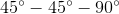triangles with the sides of the square. Using thespecial triangle ratio of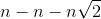, we know that if the hypotenuse isthen the length of each side must be. The area of the square is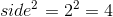.

### Example Question #5 : How To Find The Area Of A Square

One side of a square is 6 inches long.  What is the area of the square in inches?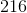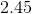Explanation:

To find the area of a square, you only need to know one side. The length of one side squared is the area.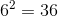### Example Question #6 : How To Find The Area Of A Square

Sidein the square shown below has a length of 13 meters. What is the total area of the square?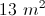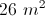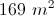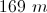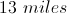Explanation:

Since the shape in question is a square, we know thatis the length of all four sides.

The formula for the area of a square is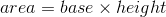.

In this case, area =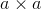, or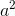.  Plug in the given value of a, 13 meters, to solve for the area: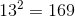Remember to use the correct units, in this square meters.

### Example Question #691 : Plane Geometry

Find the area of a square that has side lengths ofmm.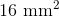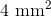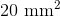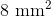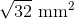Explanation:

The area of any square is: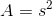, so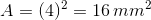### Example Question #8 : How To Find The Area Of A Square

How much more area does a square with a side of 2r have than a circle with a radius r?  Approximate  π by using 22/7.

6/7 square units

4/7 square units

1/7 square units

12/14 square units

6/7 square units

Explanation:

The area of a circle is given by A = πr2 or 22/7r2

The area of a square is given by A = s2 or (2r)2 = 4r2

Then subtract the area of the circle from the area of the square and get 6/7 square units.

### Example Question #9 : How To Find The Area Of A Square

If the perimeter of a square is 44 centimeters, what is the area of the square in square centimeters?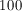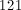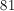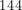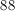Explanation:

Since the square's perimeter is 44, then each side is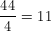.

Then in order to find the area, use the definition that the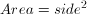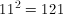### Example Question #692 : Plane Geometry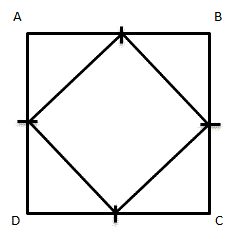Given square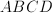, with midpoints on each side connected to form a new, smaller square.  How many times bigger is the area of the larger square than the smaller square?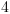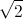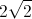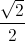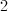Explanation:

Assume that the length of each midpoint is 1.  This means that the length of each side of the large square is 2, so the area of the larger square is 4 square units.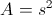To find the area of the smaller square, first find the length of each side.  Because the length of each midpoint is 1, each side of the smaller square is(use either the Pythagorean Theorem or notice that these right trianges are isoceles right trianges, so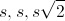can be used).

The area then of the smaller square is 2 square units.

Comparing the area of the two squares, the larger square is 2 times larger than the smaller square.

← Previous 1 3 4 5 6 7 8 9 15 16